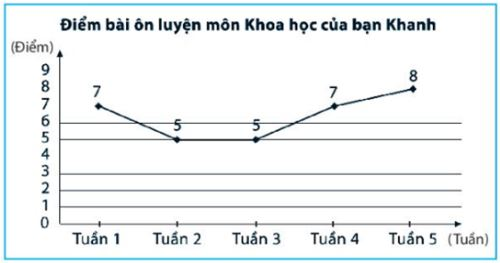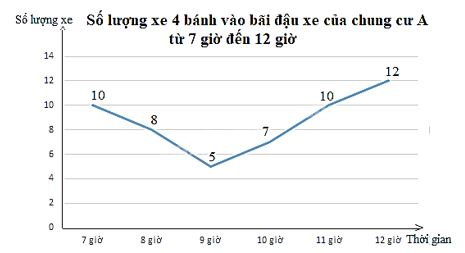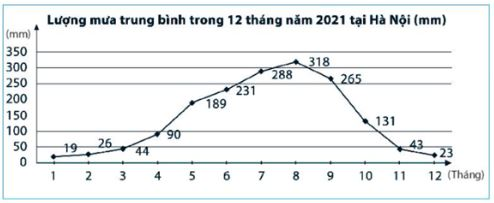## Solving SBT lesson 3 Line chart (C5 Math 7 – Horizon) – Math book

Solve SBT lesson 3 Line chart (C5 Math 7 – Horizon)
======

### Solve problem 1 page 113 SBT Math 7 Creative horizon episode 1 – CTST

Read the information from the following chart and record it in the corresponding statistics table.Detailed instructions for solving Lesson 1

Solution method

Detailed explanation

Observing the chart, we can see that the graph shows the score of Khanh’s Science review lesson in:

– Week 1 is: 7;

– Week 2 is: 5;

– Week 3 is: 5;

– Week 4 is: 7;

– Week 5 is: 8.

So we have the following table:

 Khanh’s Science review score in 5 weeks Week first 2 3 4 5 Point 7 5 5 7 8

–>

— *****

### Solution 2 page 113 SBT Math 7 Creative horizon episode 1 – CTST

Draw a graph to represent the data in the following statistical table:

 Number of 4-wheel vehicles entering the parking lot of apartment A from 7am to 12pm Hour Number of vehicles 7 o’clock ten 8 o’clock 8 9 o’clock 5 10 o’clock 7 11 o’clock ten 12 o’clock twelfth

Detailed instructions for solving Lesson 2

Solution method

To draw a straight line graph representing the statistics of the number of 4-wheel vehicles entering the parking lot of Apartment A from 7am to 12pm, we perform the following steps:

Step 1: Draw two vertical and horizontal axes at right angles to each other.

– Horizontal axis: Record the time points (7 hours, 8 hours, 9 hours, 10 hours, 11 hours, 12 hours).

– Vertical axis: Select the appropriate division for the data and write the numbers in the divisions.

Here we can choose to divide by 2.

Step 2:

– At the 7 o’clock mark on the horizontal axis, mark a point about 10 from the vertical time point at that time, corresponding to the division on the vertical axis.

The same goes for the rest of the time points.

– Draw straight lines connecting each pair of points corresponding to a pair of consecutive time points, we get a curved line representing the change of data over time.

Step 3: Finalize the chart.

– Write a name for the chart: Number of 4-wheel vehicles entering the parking lot of Apartment A from 7am to 12pm.

– Note the data values ​​at the beginning of the line segment.

– Record units on two axes.

Detailed explanation

We have the following chart:–>

— *****

### Solve problem 3 pages 113 SBT Math 7 Creative horizon episode 1 – CTST

Observe the chart below and answer the questions.(Source: https://kenhthoitiet.vn/)

a) What does the graph represent information about?

b) What is the unit of time?

c) Which month has the highest number?

d) Which month has the lowest data?

e) During what period of time does the data increase?

g) During what period of time did the data decrease?

Detailed instructions for solving Lesson 3

Solution method

Detailed explanation

a) The graph shows information about the average rainfall in 12 months of 2021 in Hanoi (unit: mm).

b) The unit of time is the month.

c) August has the highest data (318 mm).

d) January has the lowest figure (19 mm).

e) The figures increased between January and August (up from 19 mm to 318 mm).

g) Figures decrease between August and December (down from 318 mm to 23 mm)

–>

— *****